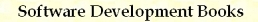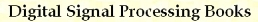Dawson integral approximations by Stanislav Sýkora, Extra Byte, Via R.Sanzio 22C, Castano Primo, Italy 20022 Published as a code-snippet in Stan's Library, Ed.S.Sykora, Vol.IV. First release February 1, 2012. Permalink via DOI: 10.3247/SL4Soft12.001
 Other programming articles in Stan's Library | Math books | Software books and Links SCIENCE Links | Stan's HUB
 This article presents a series of rational-function approximations for the Dawson integral function F(x) which exhibit correct behavior for very small and very large values of the argument. For any real argument, the worst absolute error of the best approximation presented here is about 2.1e-6, while the worst relative error is 10.0 ppm. These are not the most accurate known approximations but they are computationally extremely efficient, and perfectly sufficient for most practical purposes. Snippets of pertinent C/C++ and Matlab code are included and may be freely copied. If used in a published work, however, a link or a citation should be included, complete with the DOI of this article (see above).
 Introduction Dawson function, or Dawson integral, F(x) is an entire function [1,2,3] best defined as (1)and shown in this Figure: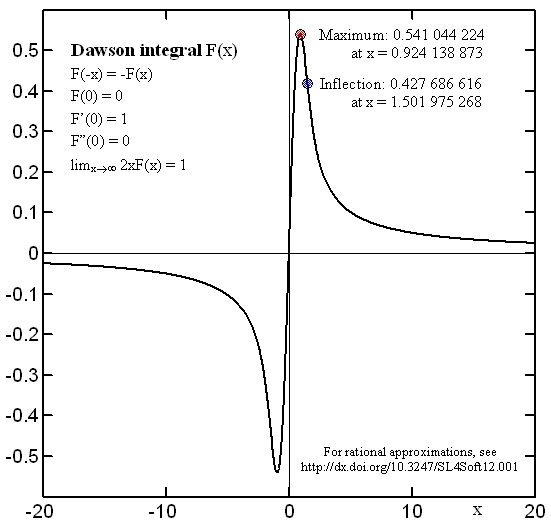It is an odd function, characterized by the following behavior [1,2,3] for very small and very large values of the argument (needles to say, these features should be respected exactly by any numeric approximation): (2)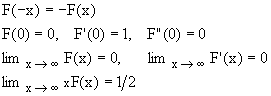Closely related [4,5,6] is the imaginary error function erfi(x), (3)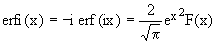whose numeric values are in fact best computed from the Dawson function: The derivatives of the Dawson function  are also best computed directly from its own values: (4)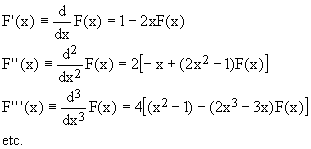The integral of F(x) is more complicated (for large x it grows as O[ln(x)]) and, if needed, should be evaluated using a different approximation. The general shape of the Dawson function resembles x/(1+x2), i.e. the "imaginary" part of a Lorentzian peak profile, except that at large values of x it tends to zero as 1/2x rather than 1/x. This fact is a good indication that an efficient and precise rational function approximation should be possible. Computation of exact values To provide a reference for the fitting of approximation parameters, as well for the respective maximum error estimates, it is necessary to be able to compute the values of the approximated function to a precision much better than that of the approximation. For sufficiently small values of the argument, F(x) can be evaluated using the well known series expansion , while for large values one can resort to the asymptotic series [3, formula 7.1.23], provided appropriate care is taken to avoid propagation of rounding errors. Another approach is to use continued fraction expansions of which the most suitable one is that of Eq.(14) in reference , due to McCabe : (5)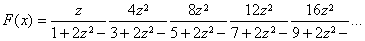Still another method to compute F(x) for any x is due to G.B.Rybicki , based on the following formula (6)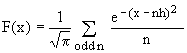which holds for arbitrary positive h and is exponentially convergent when h is small. There are of course several alternative methods [see references in 10], but they were not explored here because the scope of this study is the development of fast-executing approximations rather than a comparative evaluation of ultra-high precision evaluations. Sufficiently precise values are needed only as a reference for the optimization of numeric coefficients. The Rybicki formula had been cast into an algorithm and recommended by Recipes in C [10, chapter 6.10]. For the purposses of this work, the Recipes algorithm has been tested for maximum error and consequently modified to improve its error. The modified code, evaluated in double precision has maximum relative error below 1e-15 (compared to 5e-8 of the original version). The modification will be described in a subsequent article. In the end, the 'exact' value algorithm employed for the present work was an implementation [see ref.3, section 3.10.1] of the continued fraction of Eq.(5). It can be made arbitrarily precise and it is reasonably efficient (requires at most 30 steps to reach 15 digits precision), performs excellently for both very small and very large argument values (tested from 0 to 1000), and it is quite robust with respect to rounding errors. It's real-valued version (double) is made available here in the "C" package. Proposed approximations We approximate F(x) by rational functions of the type (7)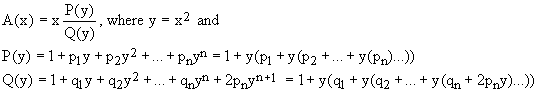which guarantee the correct behavior small and large values of x. The second forms for the polynomials P(x) and Q(x) illustrate the efficient Horner's scheme of evaluation. In total, an evaluation of n-th order approximation formula requires 2n+3 multiplications, 2n+1 additions, and one division. The value of n is called order of the approximation. The coefficients of the polynomials P(y) and Q(y) are numerically adjusted so as to minimize the maximum absolute or relative error over the interval [0,20], covered by a regular grid of 1000 test points. Details of the employed fitting algorithm will be reported elsewhere. Results The following optimal coefficients and maximum errors were obtained for various approximation orders: Order n = 1 Optimized for best absolute error:    p1 = 0.3661869354    q1 = 0.8212009121    maximum absolute error: 0.014 at x = 1.32;   (max.rel.err 6.79% at x = 2.90) Optimized for best relative error:    p1 = 0.4582332073    q1 = 0.8041350741    maximum relative error: 4.6% at x = 1.44;   (max.abs.err 2.2e-2 at x = 0.62) Order n = 2 Optimized for best absolute error:    p1 = 0.1264634385   p2 = 0.0734733435    q1 = 0.8234288542   q2 = 0.2548465663    maximum absolute error: 1.2e-3 at x = 2.36;   (max.rel.err 1.02% at x = 4.74) Optimized for best relative error:    p1 = 0.1329766230   p2 = 0.0996005943    q1 = 0.8544964660   q2 = 0.2259838671    maximum relative error: 0.52% at x = 5.15;   (max.abs.err 2.76e-3 at x = 1.06) Order n = 3 Optimized for best absolute error:    p1 = 0.1154998994   p2 = 0.0338088612   p3 = 0.0106523108    q1 = 0.7790120439   q2 = 0.3023475798   q3 = 0.0533374765    maximum absolute error: 1.19e-4 at x = 6.09;   (max.rel.err 1522 ppm at x = 6.97) Optimized for best relative error:    p1 = 0.1349423927   p2 = 0.0352304655   p3 = 0.0138159073    q1 = 0.8001569104   q2 = 0.3190611301   q3 = 0.0540828748    maximum relative error: 693 ppm at x = 3.70;   (max.abs.err 3.67e-4 at x = 0.78) Order n = 4 Optimized for best absolute error:    p1 = 0.0989636483   p2 = 0.0416374730   p3 = 0.0047331350   p4 = 0.00128928851    q1 = 0.7658933041   q2 = 0.2834457360   q3 = 0.0707918649   q4 = 0.00827315960    maximum absolute error: 1.37e-5 at x = 1.54;   (max.rel.err 165 ppm at x = 9.85) Optimized for best relative error:    p1 = 0.1107817784   p2 = 0.0437734184   p3 = 0.0049750952   p4 = 0.0015481656    q1 = 0.7783701713   q2 = 0.2924513912   q3 = 0.0756152146   q4 = 0.0084730365    maximum relative error: 90.0 ppm at x = 3.42;   (max.abs.err 4.87e-5 at x = 0.92) Order n = 5 Optimized for best absolute error:    p1 = 0.1048210880   p2 = 0.0423601431   p3 = 0.0072584274   p4 = 0.0005045886   p5 = 0.0001777233    q1 = 0.7713501425   q2 = 0.2908548286   q3 = 0.0693596200   q4 = 0.0139927487   p5 = 0.0008303974    maximum absolute error: 2.14e-6 at x = 1.42;   (max.rel.err 11.2 ppm at x = 3.38) Optimized for best relative error:    p1 = 0.1049934947   p2 = 0.0424060604   p3 = 0.0072644182   p4 = 0.0005064034   p5 = 0.0001789971    q1 = 0.7715471019   q2 = 0.2909738639   q3 = 0.0694555761   q4 = 0.0140005442   p5 = 0.0008327945    maximum relative error: 10.1 ppm at x = 1.78;   (max.abs.err 3.78e-6 at x = 0.72) Error plots The following Figures show the absolute (red) and relative (green) error curves for the rational approximations of 3rd, 4th and 5th order: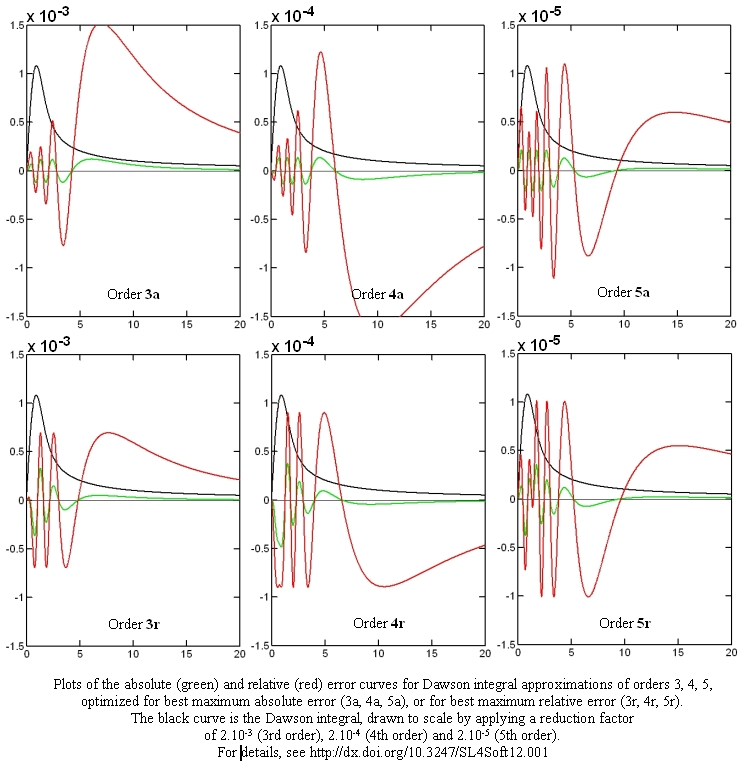Conclusions The approximations of orders 1 and 2 are probably too crude to be used in any practical application; they are listed here only for the sake of completness. However, the approximations of orders 3, 4, and 5 are certainly quite usable, offering also the possibility to struck a compromise between precision and speed (number of operations). The precision increases by a factor of almost ten from one approximation order to the next (at the cost of two more multiplications and two additions). It was not possible to go beyond order 5, though, because of numeric convergence problems encountered during the fitting of the coefficients (work in progress). The error plots 4th and 5th order approximations show small irregularities at low values of the argument, indicating that they, too, might have fitting convergence problems and, once those are overcome, a slightly better approximation coefficients might be found. It is comforting that the optimal coefficients for either absolute or relative maximum error are similar and that they vary in a regular way from one approximation order to the next one. This indicates that they are "significant" and not conditioned by an "unnatural" starting assumption or by limited precision. Likewise comforting is the fact that the maximum errors occur for moderate x values in the central part of the fitted interval rather than at its extremes, thus indicating that they are indeed the worst deviations over the whole range of real-valued arguments. The behaviour of the approximation formulas for complex arguments might be interesting but it was not yet investigated. Comparing the present approximations to others, we have on one hand brute-force ultra-precise rational Chebyshev approximations  applicable piecewise to limited segments of the full argument range which have maximum relative errors of the order of 2e-20 or better. This is very nice but neither simple, nor elegant, nor particularly performant. On the other hand, there exist very simple and fast approximations , but they are of overly limited precision, the best one having maximum relative error of 1.8e-3, worse than the order 3 approximations discussed here. The approximations presented here are of extreme simplicity, both conceptual and practical. They cover the whole range of real numbers as arguments and utilize only the four basic arithmetic operations. The formulae are easy to implement on embedded microprocessors and can be even formulated as C macros. Finally, the approximations come in several versions with precisions ranging from very crude to more than satisfactory for all imaginable practical purposes (10 ppm). The availability of the choice of the maximum allowed error may be in fact one of the principle strengths of this study. Code annex The C and Matlab codes for the used exact-value functions, and for the final approximations of orders 1 to 5 are provided below for a free download. The implemented approximations are those optimizing the relative errors. The EbDawson_C package includes a small self-standing Windows-compatible console utility to calculate the values of the Dawson function with 17 digits precision as well as all five approximations for any real argument value. EbDawson_C.zip  ... The C/C++ functions, and the console utility. EbDawson_M.zip ... The Matlab functions.
 References and Links Dawson integral on Mathworld. Dawson function on Wikipedia. Abramowitz M., Stegun I.A., Editors, Handbook of Mathematical Functions with Formulas, Graphs, and Mathematical Tables, section 7.1, 2nd Edition, Dover Publications 1972, ISBN 0-486-61272-4. Available on-line. Imaginary error function erfi(x) on Mathworld. Error function erf(x) on Mathworld. Error and related functions on Wikipedia. Sajo E., On the recursive properties of Dawson's integral, J.Phys. A: Math.Gen., 26,2977 (1993). McCabe J.H., A Continued Fraction Expansion with a Truncation Error Estimate for Dawson's Integral Math.Comput. 28, 811-816 (1974). Rybicki G.B., Computers in Physics, 3,85-87 (1989). Press W.H., Teukolsky S.A., Vetterling W.T., Flannery B.P., Numerical Recipes in C. The Art of Scientific Computing Cambridge University Press 1992. ISBN 0-521-43108-5. Chapter 6.10 can be consulted on-line. Cody W.J., Paciorek K.A., Thacher Jr. H.C., Chebyshev Approximations for Dawson Integral, Mathematics of Computation, 24,171 (1970). Lether F.G., Wenston P.R., Elementary approximations for Dawson's integral, Journal of Quantitative Spectroscopy and Radiative Transfer, 46,343-345 (1991).
 TOP | Other programming articles in Stan's Library | Math books | Software books and Links SCIENCE Links | Stan's HUB